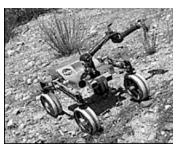Subject:
Mathematics

Topics: Probability
Common Core State Standard: S-CP.2, S-CP.7,
Concepts:

• Probability (explicit)
• Independent events (explicit)
• Variable (implicit)

Note: Note: “Explicit” refers to concepts which are named and discussed in the course of the lesson; “implicit” refers to concepts which students experience in the course of the lesson but which are not named and discussed.
Knowledge and Skills:

• Can carry out binary operations with decimals
• Can solve multi-step problems
• Can find the probability of “AND” and “OR” combinations of independent events

Lesson:

Procedure:

Distribute the handout to students working in teams of two. Ask the teams to read the first four paragraphs, then discuss, as a class, whether or not the likelihood of both motors on a side failing is greater than, equal to, or less than the likelihood of one motor failing.

Ask the teams to think of examples of the simultaneous occurrence of two events, each of which is individually unlikely, and discuss these examples until students understand why the likelihood of both events occurring will be less than the likelihood of either event occurring individually.

Review (or introduce) the formula given for finding the likelihood of the occurrence of two events together. Ensure that students understand that this is the formula for independent events, and what the word independent” means in this context. Then ask the teams to answer questions 1 and 2 (or you may choose to work through these two questions as a class). (Answer: 0.000009, or 9 out of one million)

Read the next paragraph, and ensure that students grasp its meaning. Discuss now whether or not the likelihood of either of two events occurring will be greater than, equal to, or less than the likelihood of only one of the events occurring. As before, create examples of this situation until students understand that the likelihood will be greater.

Now review (or introduce) the formula for finding the probability of either of two events occurring, and ask teams to answer question #3 (or work through it as a class). (Answer: 0.000017999919 is the exact answer, but in the real world this would be rounded to .000018.)

Finally, ask teams to find the answer to question #4. Circulate as they work, and ask questions to ensure that students understand how to use each of the two probability equations in this situation. (Answer: 0.000000053999999271, which would be rounded to .000000054.)

### Robot RoverThis Mars robot rover has four wheels. Each wheel is turned by a motor.

In order to accomplish its mission, the robot will be required to move around on the surface of Mars (starting and stopping) for 60 days.

Testing of the kinds of motors that will be used on the wheels shows that the probability that a motor will fail in 60 days of start-stop-start running is 3 out of a thousand, or 0.003.

However, if only one motor fails, the robot can keep moving. Both motors on a side must fail to stop the robot.

Remember this rule of probability for independent 1 events A and B:

Probability of both A and B = (Probability of A) × (Probability of B)

1) What is the probability of failure of both of the two motors on the right side?

2) What is the probability of failure of both of the two motors on the left side?

Our mission will fail if either #1 or #2 above occurs.

3) Use this law of probability to find out how likely that is:

Probability of either X or Y =

Probability of X + probability of Y – (Probability of X) × (Probability of Y).

4) Suppose we put three wheels on each side of the robot, so that all three motors must fail to stop the robot. What is the new probability of mission failure?

1 Note that we are assuming in this calculation that failures of the motors are independent events—that is, if a motor fails, that event has no influence on the probability that any of the other motors will fail. This may actually not be true (because if one motor fails then the other motors must work harder or may be more likely to fail), but we will ignore that for now.